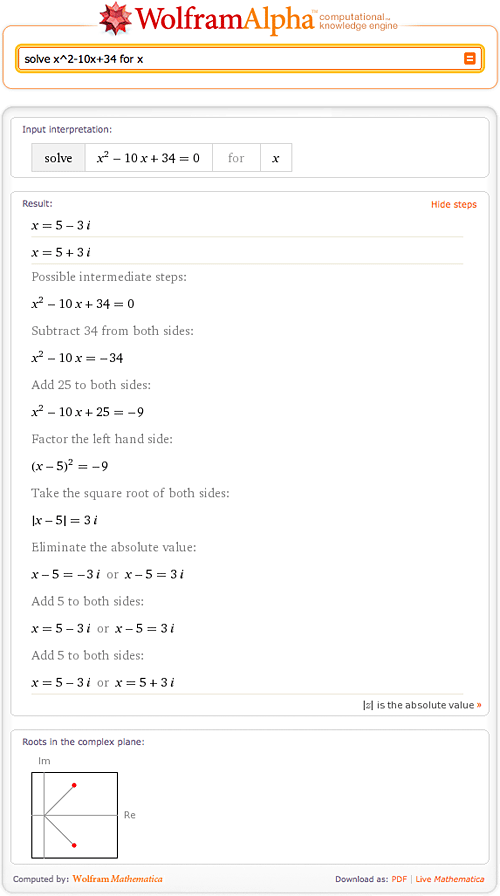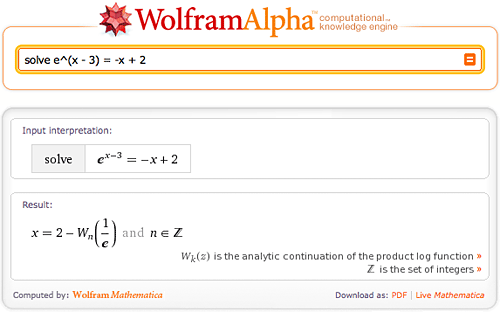# Finding Roots

March 19, 2010 —Comments Off

Steven Strogatz, a professor of applied mathematics at Cornell University, is currently blogging for The New York Times about issues “from the basics of math to the baffling”. It’s been a fascinating series, starting with preschool math and progressing through subtraction, division, complex numbers, and more. As Wolfram|Alpha is such a powerful tool for working with mathematical concepts, we thought it’d be fun to show how to use it to explore some of the topics in Strogatz’s blog.

First up is Strogatz’s post on “Finding Your Roots”. For a brief introduction to Wolfram|Alpha’s ability to find roots, try “root of 4x+2”.Here we found the one and only root of 4x+2, but what if there is more than one root? Not a problem for Wolfram|Alpha—try “4x^2 + 3x – 4”.

Of course, Wolfram|Alpha has the ability to find complex roots as well. Try “solve x^2-10x+34 for x”.Here you can see the complex roots of the quadratic function and a visual representation of the roots, as well as the steps to the solution.

You can even try to stump Wolfram|Alpha with a trig function, such as “roots of e^x*(x^3+4x-2)”.

If you want to make this a little more complicated, enter  “solve e^(x – 3) = -x + 2” into Wolfram|Alpha.Now that you have seen a few examples of what Wolfram|Alpha can do, try your hardest problem.

Plz, what is the solution of this equation:
x^(5/3)-1=0;

Posted by Tag Mohamed Amine March 19, 2010 at 6:22 pm

Sorry I need the solution of this equation:
x^(5/3)+1=0

Posted by Tag Mohamed Amine March 19, 2010 at 6:24 pm

I had a fun time playing around with this tool. A friend of mine studies informatics and I bet he’s going to love it!

Posted by warrior guide July 15, 2010 at 2:32 am

This is a great setup, I was never this deep into math but my daughter is. I like the layout, great webmaster…

Posted by Live Prayer February 10, 2012 at 2:08 am

Great examples! Would it be possible to add an example of finding a root of a trigonometric function?

Posted by Drake Cennedig October 11, 2012 at 12:52 am

Find the root of 7,396.6 to the nearest thousandth

Posted by hyren dulay December 9, 2014 at 6:47 am